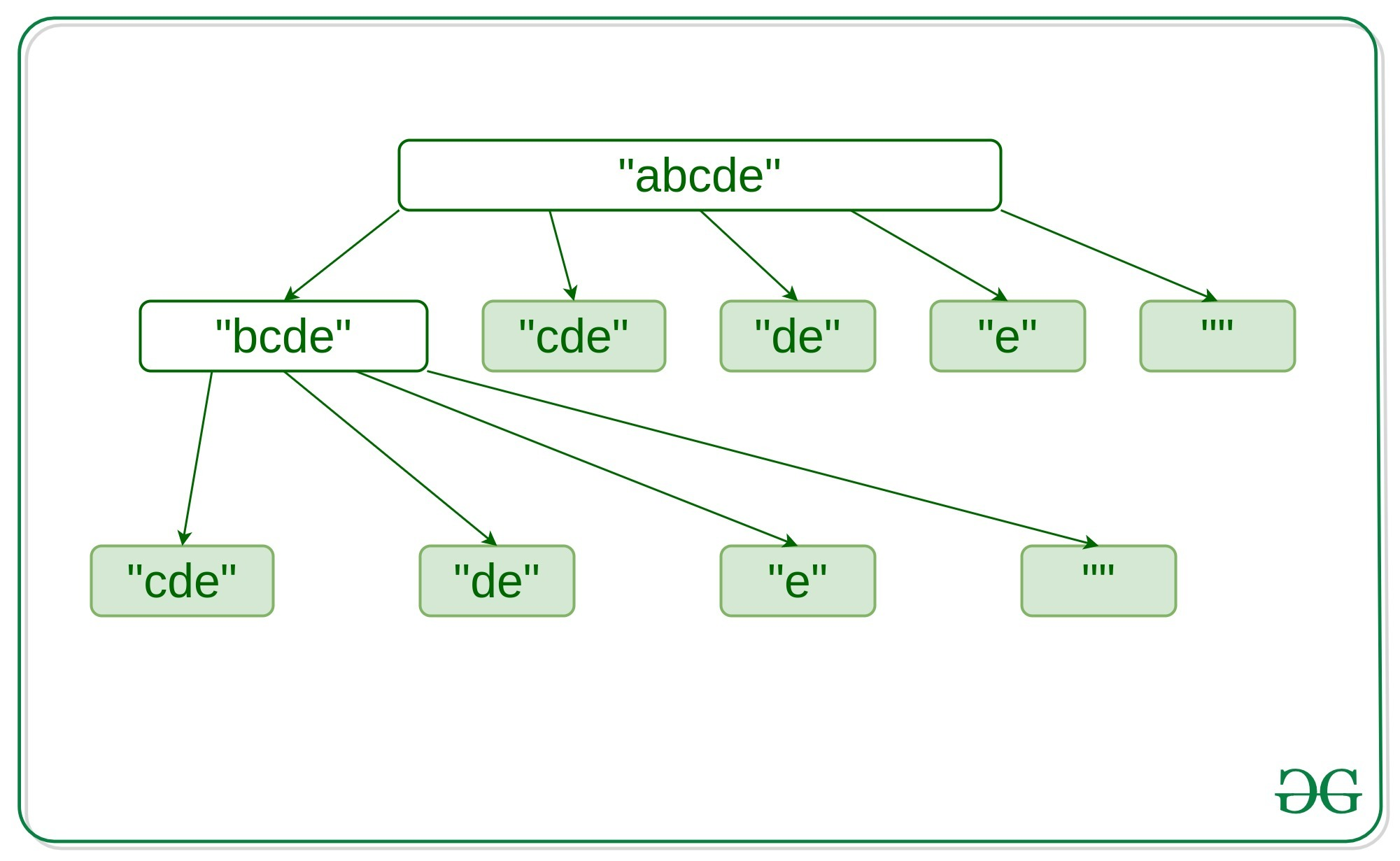# Word Break Problem | DP-32 | Set – 2

Given a non-empty sequence S and a dictionary dict[] containing a list of non-empty words, print all possible ways to break the sentence in individual dictionary words.

Examples:

Input: S = “catsanddog”
dict[] = {“cat”, “cats”, “and”, “sand”, “dog”}
Output:
“cats and dog”
“cat sand dog”

Input: S = “pineapplepenapple”
dict[] = {“apple”, “pen”, “applepen”, “pine”, “pineapple”}
Output:
“pine apple pen apple”
“pineapple pen apple”
“pine applepen apple”

## Recommended: Please try your approach on {IDE} first, before moving on to the solution.

A similar problem to this is discussed in this article, where the task is to check that is there any solution such that the sequence can be broken into the dictionary words.

Approach: The idea is to check for every substring starting from any position I , such that it ends at the length of the string which is present in the dictionary then simply recurse for the substring [0, I]. Meanwhile, store the overlapping subproblems for each substring to avoid the computation of the subproblem again. Overlapping subproblems can be shown as follows –Below is the implementation of the above approach:

## C++

 `// C++ implementation to break ` `// a sequence into the words of ` `// the dictionary ` ` `  `#include ` ` `  `using` `namespace` `std; ` ` `  `// Unordered_map used for storing ` `// the sentences the key string  ` `// can be broken into ` `unordered_map > mp; ` ` `  `// Unordered_set used  ` `// to store the dictionary. ` `unordered_set dict; ` ` `  `// Utility funtion used for  ` `// printing the obtained result ` `void` `printResult(vector A) ` `{ ` `    ``for` `(``int` `i = 0; i < A.size(); i++) ` `        ``cout << A[i] << ``'\n'``; ` `} ` ` `  `// Utility function for  ` `// appending new words  ` `// to already existing strings ` `vector combine( ` `     ``vector prev, string word){ ` `     `  `    ``// Loop to find the append string ` `    ``// which can be broken into ` `    ``for` `(``int` `i = 0; i < prev.size(); i++) { ` `        ``prev[i] += ``" "` `+ word; ` `    ``} ` `    ``return` `prev; ` `} ` ` `  `// Utility funtion for word Break  ` `vector wordBreakUtil(string s) ` `{    ` `    ``// Condition to check if the ` `    ``// subproblem is already computed ` `    ``if` `(mp.find(s) != mp.end()) ` `        ``return` `mp[s]; ` `    ``vector res; ` `     `  `    ``// If the whole word is a dictionary ` `    ``// word then directly append into  ` `    ``// the result array for the string ` `    ``if` `(dict.find(s) != dict.end()) ` `        ``res.push_back(s); ` `     `  `    ``// Loop to iterate over the substring ` `    ``for` `(``int` `i = 1; i < s.length(); i++) { ` `        ``string word = s.substr(i); ` `         `  `        ``// If the substring is present into ` `        ``// the dictionary then recurse for ` `        ``// other substring of the string ` `        ``if` `(dict.find(word) != dict.end()) { ` `            ``string rem = s.substr(0, i); ` `            ``vector prev =  ` `             ``combine(wordBreakUtil(rem), word); ` `            ``res.insert(res.end(),  ` `                 ``prev.begin(), prev.end()); ` `        ``} ` `    ``} ` `     `  `    ``// Store the subproblem  ` `    ``// into the map ` `    ``mp[s] = res; ` `    ``return` `res; ` `} ` ` `  `// Master wordBreak function converts  ` `// the string vector to unordered_set ` `vector wordBreak(string s,  ` `             ``vector& wordDict) ` `{ ` `    ``// Clear the previous stored data ` `    ``mp.clear(); ` `    ``dict.clear(); ` `    ``dict.insert(wordDict.begin(), wordDict.end()); ` `    ``return` `wordBreakUtil(s); ` `} ` ` `  `// Driver Code ` `int` `main() ` `{ ` `    ``vector wordDict1 = {  ` `        ``"cat"``, ``"cats"``, ``"and"``, ``"sand"``, ``"dog"` `}; ` `    ``printResult(wordBreak(``"catsanddog"``, wordDict1)); ` `    ``return` `0; ` `} `

Output:

```cat sand dog
cats and dog
```

Attention reader! Don’t stop learning now. Get hold of all the important DSA concepts with the DSA Self Paced Course at a student-friendly price and become industry ready.

My Personal Notes arrow_drop_upCheck out this Author's contributed articles.

If you like GeeksforGeeks and would like to contribute, you can also write an article using contribute.geeksforgeeks.org or mail your article to contribute@geeksforgeeks.org. See your article appearing on the GeeksforGeeks main page and help other Geeks.

Please Improve this article if you find anything incorrect by clicking on the "Improve Article" button below.